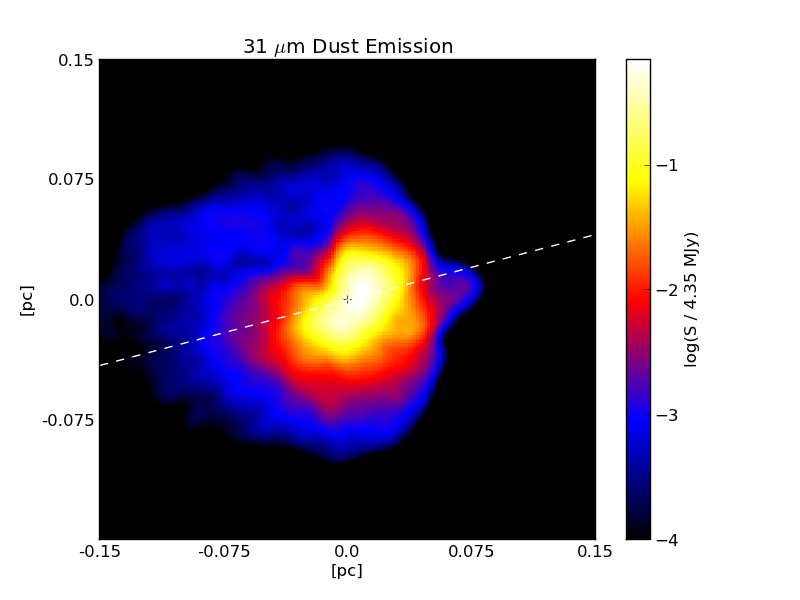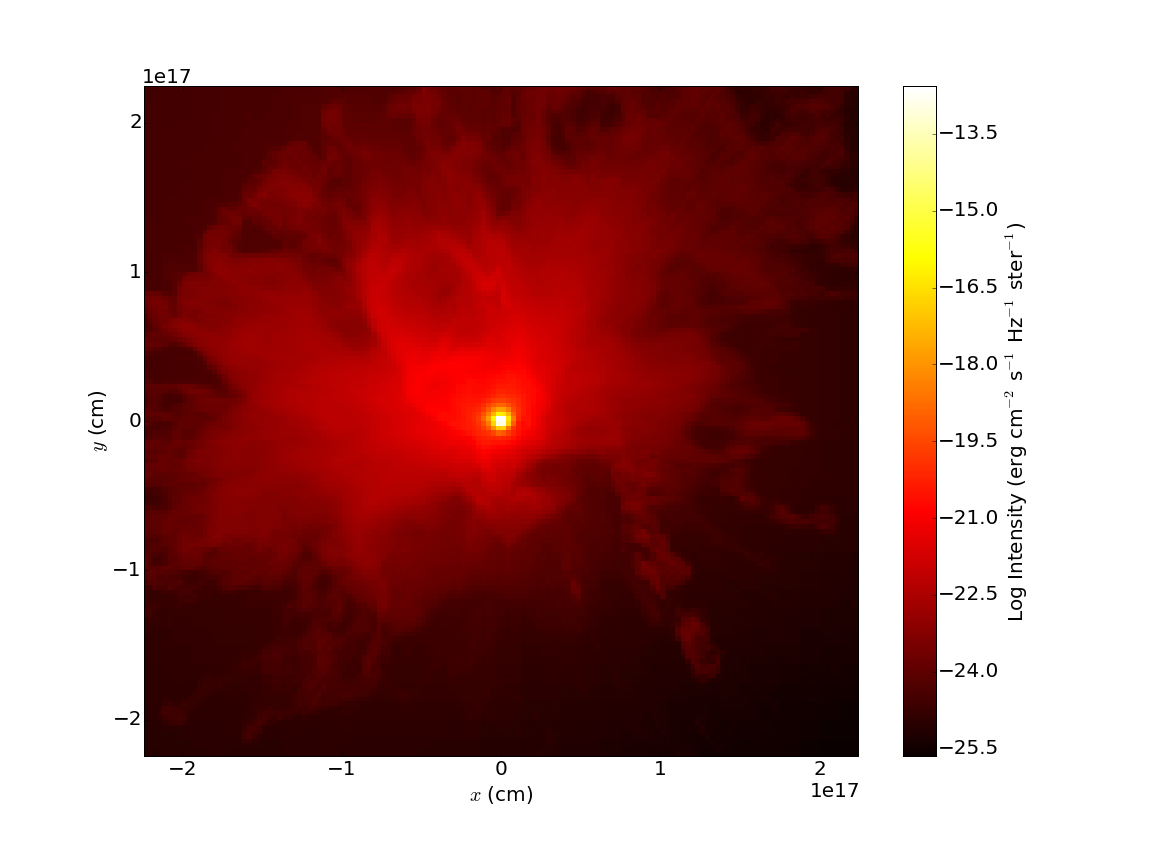New in version 2.6.Above: a sample image showing the continuum dust emission image around a massive protostar made using RADMC-3D and plotted with pyplot.

RADMC-3D is a three-dimensional Monte-Carlo radiative transfer code that is capable of handling both line and continuum emission. yt comes equipped with a `RadMC3DWriter` class that exports AMR data to a format that RADMC-3D can read. Currently, only the ASCII-style data format is supported. In principle, this allows one to use RADMC-3D to make synthetic observations from any simulation data format that yt recognizes.

## Continuum Emission¶

To compute thermal emission intensities, RADMC-3D needs several inputs files that describe the spatial distribution of the dust and photon sources. To create these files, first import the RADMC-3D exporter, which is not loaded into your environment by default:

```import yt
import numpy as np
```

Next, load up a dataset and instantiate the `RadMC3DWriter`. For this example, we’ll use the “StarParticle” dataset, available here.

```ds = yt.load("StarParticles/plrd01000/")
```

The first data file to create is the “amr_grid.inp” file, which describes the structure of the AMR index. To create this file, simply call:

```writer.write_amr_grid()
```

Next, we must give RADMC-3D information about the dust density. To do this, we define a field that calculates the dust density in each cell. We assume a constant dust-to-gas mass ratio of 0.01:

```dust_to_gas = 0.01
def _DustDensity(field, data):
return dust_to_gas * data["density"]
```

We save this information into a file called “dust_density.inp”.

```writer.write_dust_file(("gas", "dust_density"), "dust_density.inp")
```

Finally, we must give RADMC-3D information about any stellar sources that are present. To do this, we have provided the `RadMC3DSource` class. For this example, we place a single source with temperature 5780 K at the center of the domain:

```radius_cm = 6.96e10
mass_g = 1.989e33
position_cm = [0.0, 0.0, 0.0]
temperature_K = 5780.0

sources_list = [star]
wavelengths_micron = np.logspace(-1.0, 4.0, 1000)

writer.write_source_files(sources_list, wavelengths_micron)
```

The last line creates the files “stars.inp” and “wavelength_micron.inp”, which describe the locations and spectra of the stellar sources as well as the wavelengths RADMC-3D will use in it’s calculations.

If everything goes correctly, after executing the above code, you should have the files “amr_grid.inp”, “dust_density.inp”, “stars.inp”, and “wavelength_micron.inp” sitting in your working directory. RADMC-3D needs a few more configuration files to compute the thermal dust emission. In particular, you need an opacity file, like the “dustkappa_silicate.inp” file included in RADMC-3D, a main “radmc3d.inp” file that sets some runtime parameters, and a “dustopac.inp” that describes the assumed composition of the dust. yt cannot make these files for you; in the example that follows, we used a “radmc3d.inp” file that looked like:

```nphot = 1000000
nphot_scat = 1000000
```

which basically tells RADMC-3D to use 1,000,000 photon packets instead of the default 100,000. The “dustopac.inp” file looked like:

```2
1
-----------------------------
1
0
silicate
-----------------------------
```

To get RADMC-3D to compute the dust temperature, run the command:

```./radmc3D mctherm
```

in the directory that contains your “amr_grid.inp”, “dust_density.inp”, “stars.inp”, “wavelength_micron.inp”, “radmc3d.inp”, “dustkappa_silicate.inp”, and “dustopac.inp” files. If everything goes correctly, you should get a “dust_temperature.dat” file in your working directory. Once that file is generated, you can use RADMC-3D to generate SEDs, images, and so forth. For example, to create an image at 31 microns, do the command:

```./radmc3d image lambda 31 sizeau 30000 npix 800
```

which should create a file called “image.out”. You can view this image using pyplot or whatever other plotting package you want. To facilitate this, we provide helper functions that parse the image.out file, returning a header dictionary with some useful metadata and an np.array containing the image values. To plot this image in pyplot, you could do something like:

```import matplotlib.pyplot as plt
import numpy as np

x_lo = -x_hi
y_lo = -y_hi

X = np.linspace(x_lo, x_hi, Nx)
Y = np.linspace(y_lo, y_hi, Ny)

plt.pcolormesh(X, Y, np.log10(image), cmap='hot')
cbar = plt.colorbar()
plt.axis((x_lo, x_hi, y_lo, y_hi))
ax = plt.gca()
ax.set_xlabel(r"\$x\$ (cm)")
ax.set_ylabel(r"\$y\$ (cm)")
plt.savefig('dust_continuum.png')
```

The resulting image should look like:This barely scratches the surface of what you can do with RADMC-3D. Our goal here is just to describe how to use yt to export the data it knows about (densities, stellar sources, etc.) into a format that RADMC-3D can recognize.

## Line Emission¶

The file format required for line emission is slightly different. The following script will generate two files, one called “numderdens_co.inp”, which contains the number density of CO molecules for every cell in the index, and another called “gas-velocity.inp”, which is useful if you want to include doppler broadening.

```import yt

x_co = 1.0e-4
mu_h = yt.YTQuantity(2.34e-24, 'g')
def _NumberDensityCO(field, data):
return (x_co/mu_h)*data["density"]